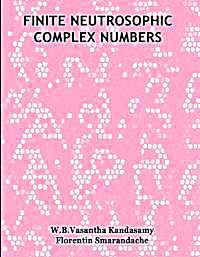#jsDisabledContent { display:none; } My Account | Register | Help

Mathematical AnalysisMathematical analysis, which mathematicians refer to simply as analysis, has its beginnings in the rigorous formulation of infinitesimal calculus. It is a branch of pure mathematics that includes the theories of differentiation, integration and measure, limits, infinite series, and analytic functions. These theories are often studied in the context of real numbers, complex numbers, and real and complex functions. However, they can also be defined and studied in any space of mathematical objects that has a definition of nearness (a topological space) or, more specifically, distance (a metric space).•Finite Neutrosophic Complex Numbers

By: Florentin Smarandache; W. B. Vasantha Kandasamy

This book is organized into 5 chapters. The first chapter introduces real neutrosophic complex numbers. Chapter two introduces the notion of finite complex numbers; algebraic structures like groups, rings etc are defined using them. Matrices and polynomials are constructed using these finite complex numbers. Chapter three introduces the notion of neutrosophic complex modulo integers. Algebraic structures using neutrosophic complex modulo integers are built and around 90 ...

In this chapter we for the first time we define the notion of integer neutrosophic complex numbers, rational neutrosophic complex numbers and real neutrosophic complex numbers and derive interesting properties related with them.

•Intro to differential equations : What is a differential equation

By: Sal Khan

What a differential equation is and some terminology.

Differential equations with only first derivatives.

•Linear homogeneous equations : 2nd Order Linear Homogeneous Differ...

By: Sal Khan

Another example with initial conditions!

Linear differential equations that contain second derivatives

•Linear homogeneous equations : 2nd Order Linear Homogeneous Differ...

By: Sal Khan

Let's find the general solution!

Linear differential equations that contain second derivatives

•Linear homogeneous equations : 2nd Order Linear Homogeneous Differ...

By: Sal Khan

Introduction to 2nd order, linear, homogeneous differential equations with constant coefficients.

Linear differential equations that contain second derivatives

•Linear homogeneous equations : 2nd Order Linear Homogeneous Differ...

By: Sal Khan

Let's use some initial conditions to solve for the particular solution

Linear differential equations that contain second derivatives

•Separable equations : Separable differential equations 2

By: Sal Khan

Another separable differential equation example.

Differential equations with only first derivatives.

•A Treatise on Differential Equations

By: Boole, George, 1815-1864; Todhunter, I. (Isaac), 1820-1884

The author's notes for a second and enlarged ed. of his Treatise on differential equations, edited after his death by I. Todhunter; List of Professor Boole's writings: p. [viii]-xi

•ON THE ERROR PROPAGATION OF SOME INTERPOLATION FORMULAS FOR SECOND...

By: Fehlberg, E

Supplemental catalog subcollection information: NASA Publication Collection; Astrophysics and Technical Documents; Error propagation of interpolation formulas for second order differential equations - error analysis

•On the resonance concept in systems of linear and nonlinear ordina...

By: Karim, R. I. I. A

Supplemental catalog subcollection information: NASA Publication Collection; Astrophysics and Technical Documents; Resonance cases for systems of linear and nonlinear differential equations

•An operational unification of finite difference methods for the nu...

By: Lomax, H

Supplemental catalog subcollection information: NASA Publication Collection; Astrophysics and Technical Documents; Operational unification of finite difference methods for numerical integration of ordinary differential equations

•An elementary treatise on the differential calculus, containing th...

By: Williamson, Benjamin, 1827-1916

Supplemental catalog subcollection information: American Libraries Collection; American University Library Collection

•Problems in differential calculus. Supplementary to a treatise on ...

By: Byerly, William Elwood, 1849-1935

Supplemental catalog subcollection information: American Libraries Collection; Historical Literature

•Elements of differential calculus

By: Bass, Edgar W. (Edgar Wales), 1843-1918

Supplemental catalog subcollection information: American Libraries Collection; Historical Literature; Distributed by the Boston Society of Natural History at the request of the Centennial Commissioner for Massachusetts.

•A short course on differential equations

By: Campbell, Donald Francis, B. 1867

Supplemental catalog subcollection information: American Libraries Collection; American University Library Collection

•A short course on differential equations

By: Campbell, Donald Francis, B. 1867

Supplemental catalog subcollection information: American Libraries Collection; American University Library Collection

•A short course on differential equations

By: Campbell, Donald Francis, B. 1867

Supplemental catalog subcollection information: Canadian Libraries Collection; Canadian University Library Collection; Candian History

•Differential equations

By: Tierney, John A., 1917

; Includes bibliographies and index

•Theory of differential equations; Volume: 4

By: Forsyth, Andrew Russell, 1858-1942

Supplemental catalog subcollection information: American Libraries Collection; Historical Literature

•Theory of differential equations; Volume: 1

By: Forsyth, Andrew Russell, 1858-1942

Supplemental catalog subcollection information: American Libraries Collection; Historical Literature